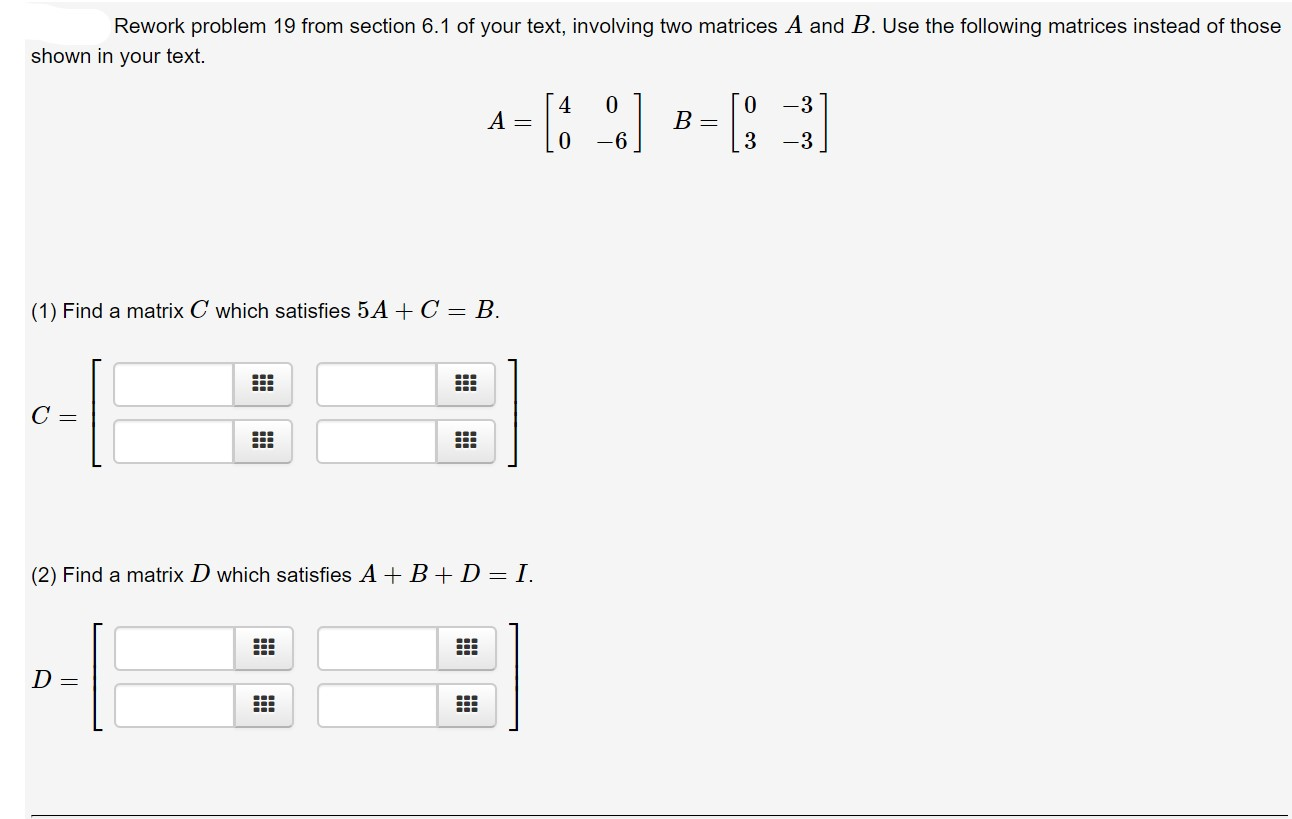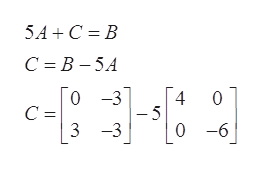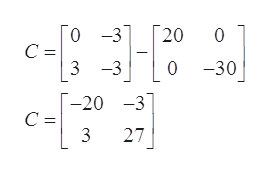# Rework problem 19 from section 6.1 of your text, involving two matrices A and B. Use the following matrices instead of thoseshown in your text.0 -30B =A =0-6-3(1) Find a matrix C which satisfies 5 A C = B.C =(2) Find a matrix D which satisfies ABIDD =

Question

?help_outlineImage TranscriptioncloseRework problem 19 from section 6.1 of your text, involving two matrices A and B. Use the following matrices instead of those shown in your text. 0 -3 0 B = A = 0 -6 -3 (1) Find a matrix C which satisfies 5 A C = B. C = (2) Find a matrix D which satisfies AB I D D = fullscreen
check_circleExpert Solution
Step 1

Given matrices are,

Step 2

(1)

Find a matrix C which satisfieshelp_outlineImage Transcriptionclose5A+C B C B-5A 0 -3 4 0 C = -5 3 -3 0 -6 fullscreen
Step 3

After solv...help_outlineImage Transcriptionclose0 -3 20 0 С - 3-3 030 -20 3 С - 3 27 fullscreen

### Want to see the full answer?

See Solution

#### Want to see this answer and more?

Solutions are written by subject experts who are available 24/7. Questions are typically answered within 1 hour*

See Solution
*Response times may vary by subject and question
Tagged in

### Math